Courses

# CPT-Nomenclature & Isomerism (Medical)

## 30 Questions MCQ Test | CPT-Nomenclature & Isomerism (Medical)

Description
This mock test of CPT-Nomenclature & Isomerism (Medical) for Class 12 helps you for every Class 12 entrance exam. This contains 30 Multiple Choice Questions for Class 12 CPT-Nomenclature & Isomerism (Medical) (mcq) to study with solutions a complete question bank. The solved questions answers in this CPT-Nomenclature & Isomerism (Medical) quiz give you a good mix of easy questions and tough questions. Class 12 students definitely take this CPT-Nomenclature & Isomerism (Medical) exercise for a better result in the exam. You can find other CPT-Nomenclature & Isomerism (Medical) extra questions, long questions & short questions for Class 12 on EduRev as well by searching above.
QUESTION: 1

Solution:
QUESTION: 2

Solution:
QUESTION: 3

### Which of the following may exist in enantiomorphs

Solution:
QUESTION: 4

Separating of d and l enantiomorphs from a racemic mixture is called

Solution:
QUESTION: 5

Which one of the following exhibits geometrical isomerism

Solution:
QUESTION: 6

The number of geometrical isomers in case of a compound with the structure  is CH- CH = CH - CH = CH - C2H5 is

Solution:
QUESTION: 7

The isomers which can be converted into another forms by rotation of the molecules around single bond are

Solution:
QUESTION: 8

The number of enantiomers of the compound CH3CHBrCHBrCO OH is

Solution:
QUESTION: 9

Reason for geometrical isomerism by 2-butene is

Solution:
QUESTION: 10

Which of the following is an chiral compound

Solution:
QUESTION: 11

Among the following the most stable compound is

Solution:
QUESTION: 12

The IUPAC name of the following compound is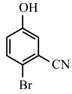Solution:
QUESTION: 13

The IUPAC name of the following compound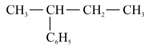is

Solution:
QUESTION: 14

The IUPAC name of neopentane

Solution:
QUESTION: 15

The correct decreasing order of priority for the functional groups of organic compounds in the IUPAC system of nomenclature is

Solution:
QUESTION: 16

The IUPAC name of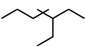is

Solution:
QUESTION: 17

The IUPAC name of the compound shown below is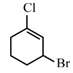Solution:
QUESTION: 18

The IUPAC name for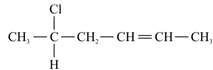is

Solution:
QUESTION: 19

What is the correct IUPAC name of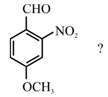Solution:
QUESTION: 20

Give the IUPAC name of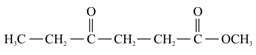Solution:
QUESTION: 21

The IUPAC name of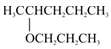Solution:
QUESTION: 22

IUPAC name of the compoundSolution:
QUESTION: 23

IUPAC name of (CH3)3CCl is

Solution:
QUESTION: 24

The IUPAC name for tertiary butyl iodide is

Solution:
QUESTION: 25

The IUPAC name of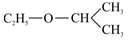Solution:
QUESTION: 26

The IUPAC name of the  (CH3)CH - CH = CH - CHOH - CH3 is

Solution:
QUESTION: 27

The IUPAC name of the compound,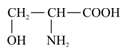Solution:
QUESTION: 28

Give the IUPAC name of the compound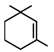Solution:
QUESTION: 29

The IUPAC name of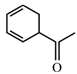is

Solution:
QUESTION: 30

The IUPAC name of  CH3 - CH = CH - C ≡ CH   is

Solution: